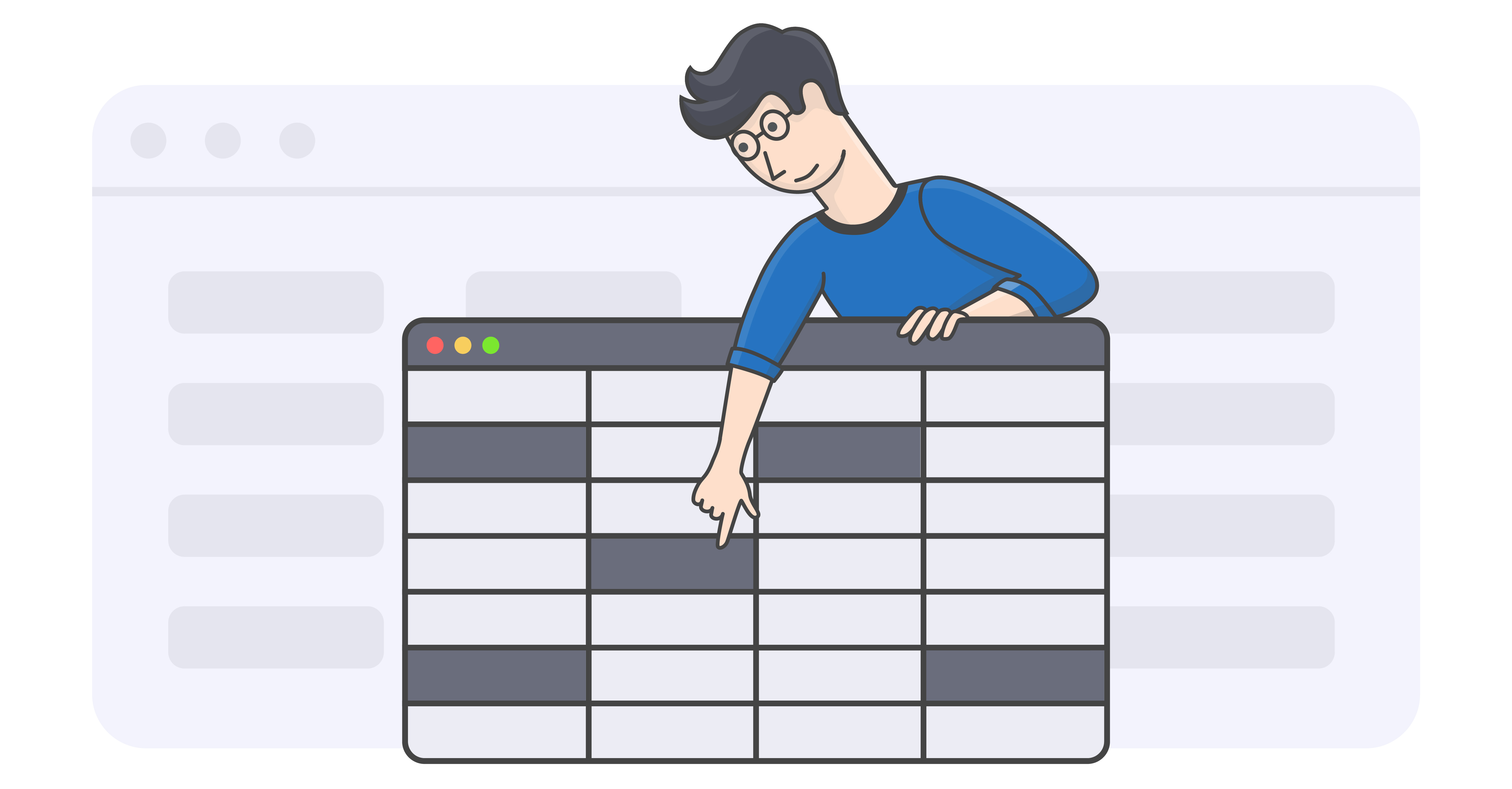Nearly all office work involves spreadsheets in one form or the other and working with them can be a challenge. Spreadsheets can get quite complicated and lack the flexibility that is provided by programming languages.

UniOffice has bridged the gap and allows you to use the flexibility of GoLang with the power of spreadsheets. You are no longer bound by the strict rules of spreadsheets and can programmatically add data into spreadsheets from databases and other data sources.

Alongside the aforementioned benefit, UniOffice also allows formatting and validating cells. Making it easy for you to perform actions such as changing the color, font size and style amongst other characteristics. You can get the values of any particular cell, row, column or the complete table and easily insert and validate excel formulas.

### Code Descriptions

In this section, we’re going to show you how to use UniOffice to implement formulas, extract data and format spreadsheets. UniOffice allows you to seamlessly work with spreadsheets and dynamically insert formulas, providing more freedom with the help of Golang.

#### Creating a New Sheet

Creating a new spreadsheet with UniOffice is a pretty straightforward process. We’ll explain it in the code playground below.

The first step is to import all the relevant packages. The fmt and log are GoLang’s inbuilt packages. Whereas, the last one is the spreadsheet package of UniOffice and provides the functions that are required for spreadsheet manipulation.

In the main function, we’re creating a spreadsheet object by the name ss and adding a sheet to it. The for loops are adding content in our newly built sheet. The outer loop is incrementally adding new rows while the inner loop adds a fixed amount of columns in each row.

After the data has been added, the sheet is being validated to root out any errors and if none exist then the simple spreadsheet is saved to a new file. The output is visible in the playground.

#### Extracting and Formatting Cells

Using UniOffice, you can extract values from existing spreadsheets and edit them however you want. Let’s edit and format the spreadsheet we created in the previous section. You can view the code and its output in the playground added below.

For this example, we’re adding in some extra packages that will be used for processing strings, adding colors and so on.

We can open an existing spreadsheet by using the powerful spreadsheet package provided by UniOffice. Since we’re using the playground, we first extract the spreadsheet data using the filestore.Open(“simple.xlsx”) and then read it by using spreadsheet.Read(file, file.Size).

To format the cells, we first create a style that we want to set to our header row. The next few lines of the code discuss how to create a style and here we are defining a simple style that sets the background color as grey.

The sheets can be extracted by using the .Sheets() function and can be iterated through using the GoLang for loop. While in the sheet, we select row 1 because we are selecting it as our header row and iterate through each of its columns and set the style.

Similarly, we iterate through each column of row and extract the cell content by using cell.GetString() and check if the string value contains a specific value. If it does then we update the value of the cell.

This code is here to just give you an overview of the power of UniOffice. You can use the functionality to perform numerous operations on your spreadsheets.

#### Inserting Formulas

We’ve talked about how to create new spreadsheets and how to edit the old ones. Now let’s move on to how we can use formulas in spreadsheets by using UniOffice. We’ll divide this section into three subsections, depending on the type of formulas we will be discussing. We’ll be creating a new excel sheet containing numeric data to implement the formulas.

##### Statistical Functions

First up are the most commonly used statistical functions. We’ll be implementing Average, Variance and Covariance formulas.

We are creating a new sheet and filling ten rows with dummy data by iterating through a for loop. Supposedly, the data is showing the number of particular products sold.

To calculate the average, we create a new row and insert two cells. The first cell contains the name of the calculation, which is Average in this case and the second cell contains the function to calculate the average.

To calculate the variance of sales of products, we create a new row and add the variance formula. This result will show us how much the results tend to vary from the mean.

The playground shows how we can calculate the variance of given cells. You can insert any formula using the command .SetFormulaRaw(“Formula”).

We can also calculate the Covariance of the sale of products in a similar fashion. Just create another row and add in the formula. We’ll skip it for now and move on to other formulas.

##### Financial Functions

Let’s test out some financial functions, for which we’ll create a new sheet by the name of financial.xlsx.

Here’s the playground example for it:

We’re creating an excel sheet similar to how we did in the previous section. We’re going to calculate the Net Present Value of our investment. We have created data that presents our investment over 5 periods and the corresponding cash flow.

The required percentage of return has been defined in cell F2 and NPV is calculated at cell B12.

As clear from the code, you can add values and position them conveniently using the UniOffice library. It seems like we’re not doing too good on our investment.

### Conclusion

In this tutorial, we learned how to play around with spreadsheets and implement different formulas. We have a diverse set of examples on our GitHub repo, feel free to check them out. If you feel that another example is needed, please open an issue on the examples repository.